Modeling Powerbus and Cable with HFSS (version 11.1.3)

 Geometry and setup Double-sided PCB: Size: 125 mm × 100 mm × 1 mm Top and bottom metal: PEC Dielectric: FR4, εr = 4.5, dielectric loss tangent = 0.015 Cable (round cable): Length: 1000 mm Radius: 2 mm Material: PEC Excitation: Voltage source (1 V, 50 ohms) Boundary: Radiation, Infinite groundAnalysis Setup: Solution Frequency: 300 MHz (<300 MHz), 1.8 GHz (>300 MHz) Maximum Number of Passes: 50 Maximum ΔS: 0.01 Do Lambda Refinement: 0.3 Maximum Refinement Passes: 20% Sweep: Sweep type: Discrete Frequency Setup: 10 MHz - 2 GHz, Step Size = 10 MHz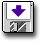hfss_powerbus_cable.zip Simulation result Simulation Time: 11 mins(< 300 MHz) + 39 mins (> 300 MHz)Number of passes completed: 7Number of tetrahedra: 10961 Decisions the user must make that affect the accuracy of the result Infinite ground plane: assign the bottom face of the radiation boundary to infinite ground plane with perfect E boundary Location of absorbing boundary: for low frequencies (<300 MHz), use a large radiation boundary (radius = 1562.5 mm). For high frequencies (>300 MHz), use a small radiation boundary (radius = 212.5 mm). Maximum ΔS: default = 0.02, this model = 0.01 Do lambda refinement: default = 0.333, this model =0.3 Cable model: use flat ribbon instead of round cable Comments How did the location of the absorbing boundary affect the result at low frequencies? Two meshes were used to model this problem. At high frequencies (> 300 MHz), the ground plane had a diameter of 212.5 mm, and was terminated at the absorbing boundary. At low frequencies (< 300 MHz), the ground plane had a diameter of 1562.5 mm in order to put the absorbing boundary sufficiently far from the object being modeled. More information ... How did we define the wire? We used a flat ribbon to model the 1-m cable. HFSS also allows us to model the cable as a round wire. More information ...
 Screen shots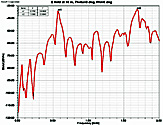Fig. 3. Electric field at 10 m, θ=0°, φ=0°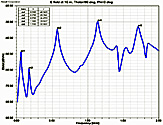Fig. 4. Electric field at 10 m, θ=90°, φ=0°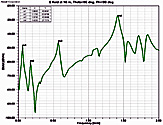Fig. 5. Electric field at 10 m, θ=90°, φ=90°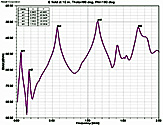Fig. 6. Electric field at 10 m, θ=90°, φ=180°